# A Question about Series-Parallel Circuits

• Engineering
Hello Guys !!

In This photos i found that
in (a) : RT= 4 Ω+ 10 Ω+ 4 Ω= 18 Ω
and
in (d) : RT= 10 Ω

but i can't understand why in (a) the total resistance equals to 18 and how will the current would pass ??

and in (d) i can't understand why the total resistance equals to 10 and why we didn't take the two 4 ohm resistances in the count ?!!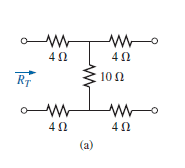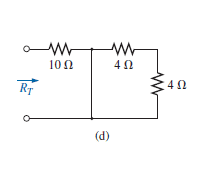Thank you Guys !!Current can flow only in close loops so for example A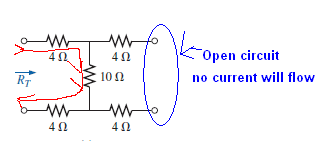In circuit D you have a short wire. And all current will flow through shorted wire.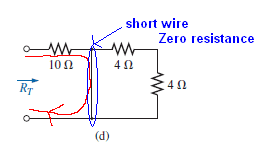#### Attachments

Current can flow only in close loops so for example A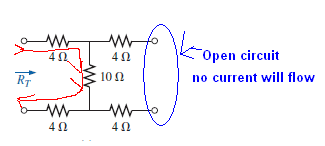In circuit D you have a short wire. And all current will flow through shorted wire.why not R=4+4=8Ω ??

#### Attachments

• untitled.bmp
184.7 KB · Views: 339
Integral
Staff Emeritus